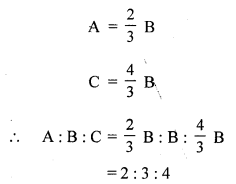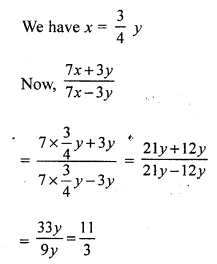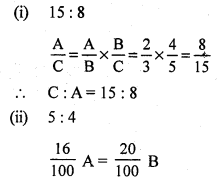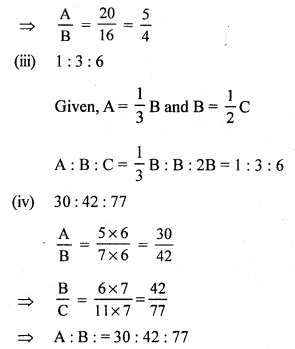## RS Aggarwal Class 7 Solutions Chapter 8 Ratio and Proportion CCE Test Paper

These Solutions are part of RS Aggarwal Solutions Class 7. Here we have given RS Aggarwal Solutions Class 7 Chapter 8 Ratio and Proportion CCE Test Paper.

Other Exercises

Question 1.
Solution:Question 2.
Solution:
The sum of ratio terms is = 2 + 3 + 5 = 10
Then, we have :
A’s share = ₹ $$\frac { 2 }{ 10 }$$ x 1100 = ₹ 220
B’s share = ₹ $$\frac { 3 }{ 10 }$$ x 1100 = ₹ 330
C’s share = ₹ $$\frac { 5 }{ 10 }$$ x 1100 = ₹ 550

Question 3.
Solution:
Product of the extremes = 25 x 6 = 150
Product of the means = 36 x 5 = 180
The product of the extremes is not equal to that of the means.
Hence, 25, 36, 5 and 6 are not in proportion.

Question 4.
Solution:
x : 18 :: 18 : 108
⇒ x x 108 = 18 x 18
(Product of extremes = Product of means)
⇒ 108x = 324
⇒ x = 3
Hence, the value of x is 3.

Question 5.
Solution:
Suppose that the numbers are 5x and 7x
Then, 5x + 7x = 84
12x = 84
x = 7
Hence, the numbers are (5 x 7) = 35 and (7 x 7) = 49.

Question 6.
Solution:
Suppose that the present ages of A and B are 4x yrs and 3x yrs, respectively
Eight years ago, age of A = (4x – 8) yrs
Eight years ago, age of B = (3x – 8) yrs
Then,
(4x – 8) : (3x – 8) = 10 : 7
⇒ $$\frac { 4x – 8 }{ 3x – 8 }$$ = $$\frac { 10 }{ 7 }$$
⇒ 28x – 56 = 30x – 80
⇒ 2x = 24
⇒ x = 12

Question 7.
Solution:
Distance covered in 60 min = 54 km
Distance covered in 1 min = $$\frac { 54 }{ 60 }$$ km
Distance covered in 40 min = $$\frac { 54 }{ 60 }$$ x 40 = 36 km

Question 8.
Solution:
Suppose that the third proportional to 8 and 12 is x
Then, 8 : 12 :: 12 : x
⇒ 8x = 144 (Product of extremes = Product of means)
x = 18
Hence, the third proportional is 18.

Question 9.
Solution:
40 men can finish the work in 60 days
1 man can finish the work in 60 x 40 days [Less men, more days]
75 men will finish the work in = $$\frac { 60 x 40 }{ 75 }$$ = 32 days
Hence, 75 men will finish the same work in 32 days.

Mark (✓) against the correct answer in each of the following :
Question 10.
Solution:
(d) 6 : 4 : 3
A = $$\frac { 3 }{ 2 }$$ B
C = $$\frac { 3 }{ 4 }$$ B
A : B : C = $$\frac { 3 }{ 2 }$$ B : B : $$\frac { 3 }{ 4 }$$ B
= 6 : 4 : 3

Question 11.
Solution:
(a) 2 : 3 : 4Question 12.
Solution:
(c) 11 : 3Question 13.
Solution:
(a) 3
Let us assume that the number to be subtracted is x
Then, (15 – x) : (19 – x) = 15 : 3
⇒ $$\frac { 15 – x }{ 19 – x }$$ = $$\frac { 3 }{ 4 }$$
⇒ 60 – 4x = 57 – 3x
⇒ x = 3

Question 14.
Solution:
(b) ₹ 360
Sum of the ratio terms = 4 + 3 = 7
B’s share = ₹ 840 x $$\frac { 3 }{ 7 }$$ = ₹ 360

Question 15.
Solution:
(c) 40 years
Suppose that the present ages of A and B are 5x yrs and 2x yrs, respectively
After 5 years, the ages of A and B will be (5x + 5) yrs and (2x + 5) yrs, respectively
Then, (5x+ 5) : (2x + 5) = 15 : 7
⇒ $$\frac { 5x+ 5 }{ 2x + 5 }$$ = $$\frac { 15 }{ 7 }$$
Cross multiplying; we get:
⇒ 35x + 35 = 30x + 75
⇒ 5x = 40
⇒ x = 8
Then, the present age of A is 5 x 8 = 40 yrs.

Question 16.
Solution:
(a) 896
Suppose that the number of boys in the school is x
Then, x : 320 = 9 : 5
⇒ 5x = 2880
⇒ x = 576
Hence, total strength of the school = 576 + 320 = 896

Question 17.
Solution:Question 18.
Solution:
(i) True
Suppose that the men proportional is x
Then, 0.4 : x :: x : 0.9
⇒ 0.9 x 0.4 = x x x (Product of extremes = Product of means)
⇒ x² = 0.36
⇒ x = 0.6
(ii) False
Suppose that the third proportional is x.
Then, 9: 12 :: 12 : x
⇒ 9x = 144 (Product of extremes = Product of means)
⇒ x = 16
(iii) True
8 : x :: 48 : 18
⇒ 144 = 48x (Product of extremes = Product of means)
⇒ x = 3
(iv) TrueHope given RS Aggarwal Solutions Class 7 Chapter 8 Ratio and Proportion CCE Test Paper are helpful to complete your math homework.

If you have any doubts, please comment below. Learn Insta try to provide online math tutoring for you.